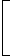# numbers aptitude questions

111. If (64)2 – (36)2 = 20 x x, then x = ?
 A. 70 B. 120 C. 180 D. 140 E. None of these

Explanation:

20 x x = (64 + 36)(64 – 36) = 100 x 28x = 100 x 28 = 140 20

112. Which one of the following can’t be the square of natural number ?
 A. 32761 B. 81225 C. 42437 D. 20164 E. None of these

Explanation:

The square of a natural number never ends in 7.42437 is not the square of a natural number.

113. (22 + 42 + 62 + … + 202) = ?
 A. 770 B. 1155 C. 1540 D. 385 x 385

Explanation:

(22 + 42 + 62 + … + 202) = (1 x 2)2 + (2 x 2)2 + (2 x 3)2 + … + (2 x 10)2

= (22 x 12) + (22 x 22) + (22 x 32) + … + (22 x 102)

= 22 x [12 + 22 + 32 + … + 102]Ref: (12 + 22 + 32 + … + n2) = 1 n(n + 1)(2n + 1)6

 =4 x 1 x 10 x 11 x 216

= (4 x 5 x 77)

= 1540.

114.

 854 x 854 x 854 – 276 x 276 x 276 = ? 854 x 854 + 854 x 276 + 276 x 276
 A. 1130 B. 578 C. 565 D. 1156 E. None of these

Explanation:

 Given Exp. = (a3 – b3) = (a – b) = (854 – 276) = 578 (a2 + ab + b2)
115. 35 + 15 x 1.5 = ?
 A. 85 B. 51.5 C. 57.5 D. 5.25 E. None of these

116. The sum of first 45 natural numbers is:
 A. 1035 B. 1280 C. 2070 D. 2140

Explanation:

Let Sn = (1 + 2 + 3 + … + 45)

This is an A.P. in which a = 1, d = 1, n = 45 and l = 45Sn = n (a + l) = 45 x (1 + 45)   = (45 x 23)   = 1035 2 2

Required sum = 1035.

117. 666 ÷ 6 ÷ 3 = ?
 A. 37 B. 333 C. 111 D. 84 E. None of these

Explanation:

 Given Exp. = 666 x 1 x 1 = 37 6 3

118. The sum of all two digit numbers divisible by 5 is:
 A. 1035 B. 1245 C. 1230 D. 945 E. None of these

Explanation:

Required numbers are 10, 15, 20, 25, …, 95

This is an A.P. in which a = 10, d = 5 and l = 95.

tn = 95a + (n – 1)d = 9510 + (n – 1) x 5 = 95(n – 1) x 5 = 85(n – 1) = 17n = 18Requuired Sum = n (a + l) = 18 x (10 + 95)   = (9 x 105)   = 945. 2 2

119. The difference between the place values of two sevens in the numeral 69758472 is
 A. 0 B. 6993 C. 699930 D. None of these

Explanation:

Required difference = (700000 – 70) = 699930

120. On dividing a number by 68, we get 269 as quotient and 0 as remainder. On dividing the same number by 67, what will the remainder ?
 A. 0 B. 1 C. 2 D. 3

Explanation:

```Number = 269 x 68 + 0 = 18292

67) 18292 (273
134
----
489
469
----
202
201
---
1
---

Therefore, Required remainder = 1```